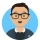Home
IT Knowledge
Inspiration
Languages
EN

# JavaScript - how to add new function to array class / type

0 points
Created by:Krzysiek
351

In this article, we would like to show you how to add a new function to the array class in JavaScript.

Below examples present how to add new properties and methods to the Array() object so we can use it on every array as it was a built-in function.

Note:

When constructing a method all arrays will have access to this method.

Custom sum numbers in array function example:

``````// ONLINE-RUNNER:browser;

Array.prototype.mySum = function() {
const sum = (accumulator, number) => accumulator + number;
return this.reduce(sum);
};

var numbers = [1, 2, 3];
console.log(numbers.mySum());  // 6``````

Custom increment numbers in array function example:

``````// ONLINE-RUNNER:browser;

Array.prototype.increment = function() {
let tmpArray = [];
for (let i = 0; i < this.length; i++) {
tmpArray[i] = this[i] + 1;
}
return tmpArray;
};

var numbers = [1, 2, 3];
console.log(numbers.increment());  // [2,3,4]``````